## Predicting income brackets

Data: Income data from the UCI ML Repository
Techniques: Classification, random forest

Here I build quick models (using sklearn and H2O) to determine income bracket from imbalanced data- the income levels are binned at below 50k and above 50k.

Some of the features are:

Age
Marital Status
Income
Family Members
No. of Dependents
Tax Paid
Investment (Mutual Fund, Stock)
Return from Investments
Education
Spouse Education
Nationality
Occupation
Region in US
Race
Occupation category

``````from collections import defaultdict
from operator import itemgetter
import numpy as np
import pandas as pd
import sklearn as sk
from sklearn import metrics
from sklearn.model_selection import StratifiedShuffleSplit
from sklearn.preprocessing import StandardScaler, Imputer
from sklearn.model_selection import train_test_split, cross_val_score, GridSearchCV
from sklearn.metrics import roc_auc_score, confusion_matrix
from sklearn.ensemble import RandomForestClassifier
from h2o.estimators.random_forest import H2ORandomForestEstimator
from h2o.model.metrics_base import H2OBinomialModelMetrics
import h2o
import os
import sys
sys.path.append('../MLRecipes')

# machine learning helper code
import ml_helper as mlhelp

%matplotlib inline
import matplotlib.pyplot as plt
import matplotlib.font_manager
from matplotlib import rcParams
import seaborn as sns
sns.set_style("whitegrid")
sns.set_context("poster")

print('Python version: %s.%s.%s' % sys.version_info[:3])
print('numpy version:', np.__version__)
print('pandas version:', pd.__version__)
print('scikit-learn version:', sk.__version__)
``````
``````Python version: 3.5.2
numpy version: 1.11.1
pandas version: 0.18.1
scikit-learn version: 0.18.1
``````
``````# Preprocessing functions
def label_map(y):
"""Encodes labels"""

# For incomes above 50k
if y == 50000:
return 1
elif y == '50000+.':
return 1
elif y == ' 50000+.':
return 1

# For incomes below 50k
elif y == -50000:
return 0
elif y == '-50000':
return 0

def isNan(num):
"""Test for Nan"""
return num != num

"""Add column for missing categorical data"""
for col in col_list:
df[col+'_missing'] = df[col].apply(isNan)
``````

#### Data preprocessing

``````train = pd.read_csv('train.csv')

``````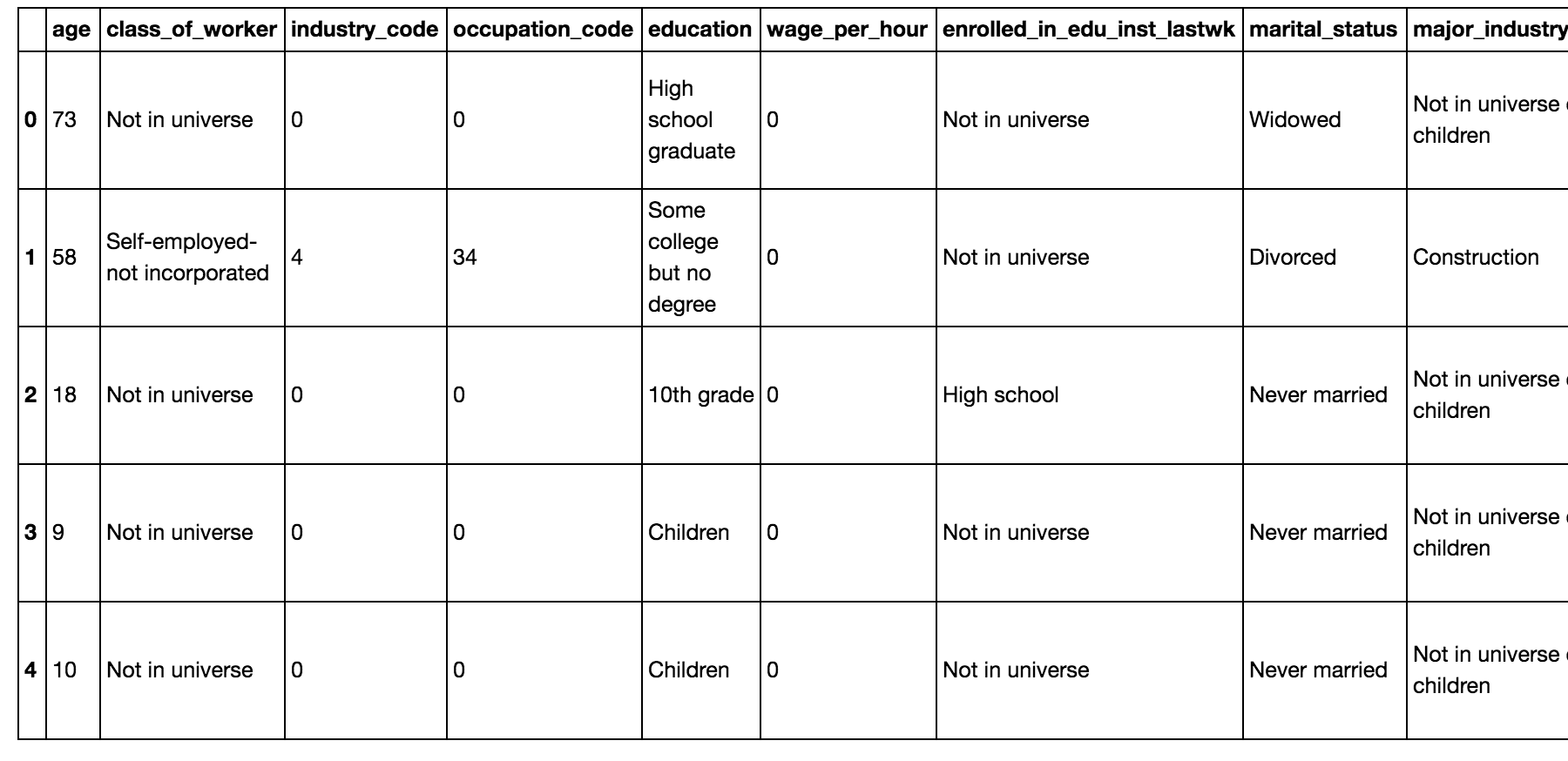``````train.shape
``````
``````(199523, 41)
``````
``````test.shape
``````
``````(99762, 41)
``````

The train data has 199,523 rows and 41 columns. The test data has 99,762 rows and 41 columns.

#### Cleanup

Let’s look at the labels:

``````train.income_level.value_counts()
``````
``````-50000    187141
50000     12382
Name: income_level, dtype: int64
``````
``````test.income_level.value_counts()
``````
``````-50000      93576
50000+.     6186
Name: income_level, dtype: int64
``````

Note: We have imbalanced classes here. The higher income class is about 6% of the total.

The labels are not written the same way. I’ll encode these variables as 0 and 1:

``````test['income_level'] = test['income_level'].apply(label_map)
train['income_level'] = train['income_level'].apply(label_map)
``````
``````# Going to combine train and test from this dataset for now
alldata = train.append(test)
``````
``````alldata.income_level.value_counts()
``````
``````0    280717
1     18568
Name: income_level, dtype: int64
``````

#### Check for missing values

``````alldata.isnull().values.any()
``````
``````True
``````

There are some missing values.

``````alldata.isnull().sum()
``````
``````age                                     0
class_of_worker                         0
industry_code                           0
occupation_code                         0
education                               0
wage_per_hour                           0
enrolled_in_edu_inst_lastwk             0
marital_status                          0
major_industry_code                     0
major_occupation_code                   0
race                                    0
hispanic_origin                       874
sex                                     0
member_of_labor_union                   0
reason_for_unemployment                 0
full_parttime_employment_stat           0
capital_gains                           0
capital_losses                          0
dividend_from_Stocks                    0
tax_filer_status                        0
region_of_previous_residence            0
state_of_previous_residence           708
d_household_family_stat                 0
d_household_summary                     0
migration_msa                       99696
migration_reg                       99696
migration_within_reg                99696
live_1_year_ago                         0
migration_sunbelt                   99696
num_person_Worked_employer              0
family_members_under_18                 0
country_father                       6713
country_mother                       6119
country_self                         3393
citizenship                             0
veterans_benefits                       0
weeks_worked_in_year                    0
year                                    0
income_level                            0
dtype: int64
``````

All the missing values are part of categorical features. I’ll fill in the nans with “missing”- then when I do one-hot encoding this will essentially create an extra “missing” category.

Note: For H2O model implementations, we don’t have to fill in these nans, but we do for sklearn’s.

``````# Have to fill in nans for sklearn implementations
alldata_nonan = alldata.fillna(value='missing')
``````

#### Explore data

``````sns.set(style='whitegrid',context='notebook')
cols=['age','wage_per_hour', 'capital_gains','capital_losses','dividend_from_Stocks' ,'d_household_family_stat','income_level']
sns.pairplot(alldata_nonan[cols],size=2.5)
plt.show()
``````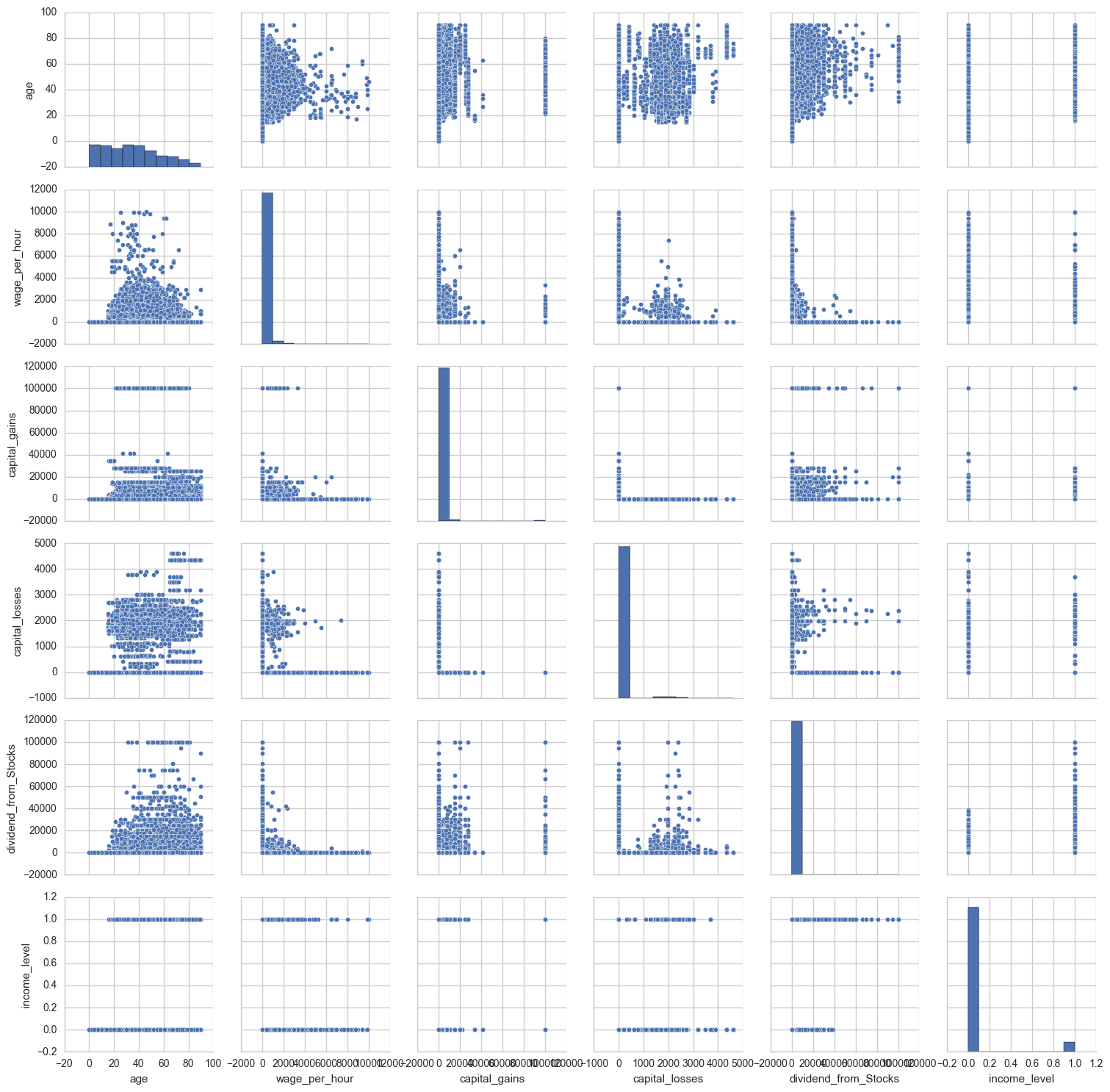The lower income bracket has lower dividend_from_stocks. Unsurprisingly, none of the youngest people (under age 20) were in the higher income bracket. Interestingly wage_per_hour is not necessarily higher for the higher income bracket.

#### Quick random forest model

I’ll do a quick model for now before doing any feature engineering, etc.

``````col_list = list(alldata_nonan)
features = mlhelp.make_normal_features(alldata_nonan, col_list)

column_to_predict = 'income_level'

# Prepare data to be used in the model by transforming
# the lists of feature-value dictionaries to vectors
# When feature values are strings, the DictVectorizer will do a binary one-hot coding
X, y, dv, mabs = mlhelp.create_data_for_model(alldata_nonan, features, column_to_predict)

(X_train, X_test, y_train, y_test) = train_test_split(X, y, test_size=0.2,
stratify=y, random_state=2016)
``````
``````rf = RandomForestClassifier(class_weight='balanced')
rf.fit(X_train, y_train)

y_predict = rf.predict(X_test)
mlhelp.classification_accuracy(y_truth=y_test, y_predict=y_predict);
``````
``````Percentage correct predictions = 99.37
Percentage correct predictions (true class 0) = 99.99
Percentage correct predictions (true class 1) = 89.93
``````
``````confmat = confusion_matrix(y_true=y_test, y_pred=y_predict)

fig,ax = plt.subplots(figsize=(2.5,2.5))
ax.matshow(confmat, cmap=plt.cm.Blues, alpha=0.3)
for i in range(confmat.shape):
for j in range(confmat.shape):
ax.text(x=j,y=i,
s=confmat[i,j],
va='center', ha='center')
plt.xlabel('predicted label')
plt.ylabel('true label')
plt.show()
``````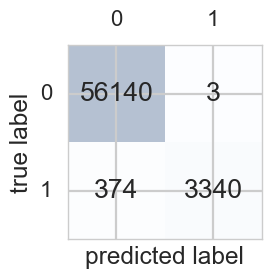The classifier does a bit worse with class 1 (the rare class).
Some important features:

``````mlhelp.print_features_importances(rf, dv, max_print=10)
``````
``````income_level 0.322192086021
num_person_Worked_employer 0.063498787871
occupation_code 0.0590363279715
weeks_worked_in_year 0.0523350224565
dividend_from_Stocks 0.0484704052559
age 0.0371623762101
industry_code 0.024954228483
major_occupation_code=Not in universe 0.022879498577
capital_gains 0.019464985337
family_members_under_18=Not in universe 0.0193774196446
tax_filer_status=Joint both under 65 0.0157218318548
``````

#### Random forest (H2O)

The H2O implementation lets you work with categorical variables well (without one-hot encoding). You can also feed in missing values.

``````h2o.init(max_mem_size = "2G", nthreads=-1)
``````
``````h2ofr = h2o.H2OFrame(alldata)
``````
``````Parse progress: |█████████████████████████████████████████████████████████| 100%
``````
``````h2ofr['income_level'] = h2ofr['income_level'].asfactor()
``````
``````splits = h2ofr.split_frame(ratios=[0.7, 0.15], seed=1)
train = splits
valid = splits
test = splits
``````
``````y = 'income_level'
x = list(h2ofr.columns)

x.remove(y) # remove response variable
``````
``````RF = H2ORandomForestEstimator(balance_classes=True)
RF.train(x=x, y=y, training_frame=train)
``````
``````drf Model Build progress: |███████████████████████████████████████████████| 100%
``````
``````RF_perf = RF.model_performance(test)
print(RF_perf)
``````
``````ModelMetricsBinomial: drf
** Reported on test data. **

MSE: 0.03933510927017885
RMSE: 0.1983308076678428
LogLoss: 0.13454365044271357
Mean Per-Class Error: 0.12912999956069804
AUC: 0.9419047339083383
Gini: 0.8838094678166766
Confusion Matrix (Act/Pred) for max f1 @ threshold = 0.15341475654483194:
``````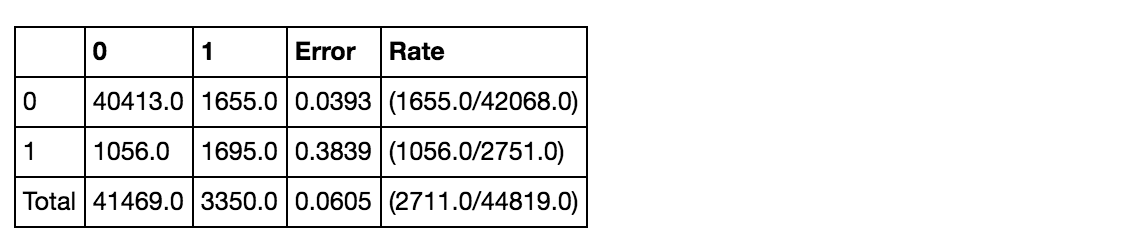``````Maximum Metrics: Maximum metrics at their respective thresholds
``````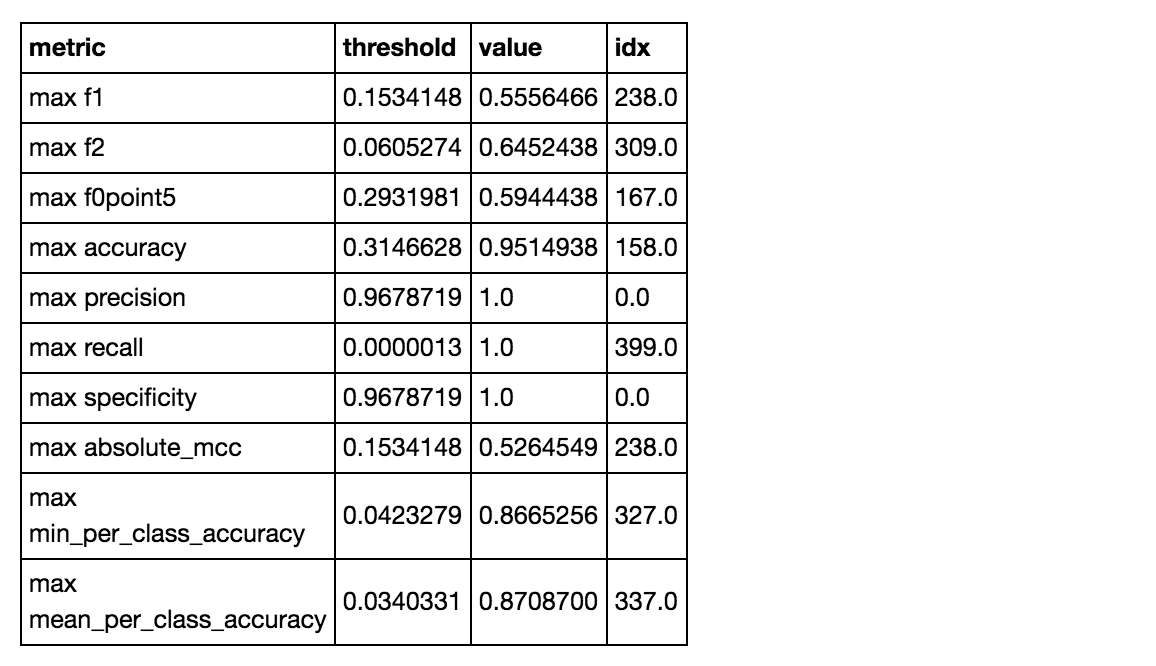``````Gains/Lift Table: Avg response rate:  6.14 %
``````

This one actually performs worse in predicting class 1 compared to the sklearn implementation. It only gets about 62% of class 1 right compared to getting 96% of class 0 right.